# 1.5. 随机梯度下降

SGD 已成功应用于在文本分类和自然语言处理中经常遇到的大规模和稀疏的机器学习问题。对于稀疏数据，本模块的分类器可以轻易的处理超过 10^5 的训练样本和超过 10^5 的特征。

• 高效。
• 易于实现 (有大量优化代码的机会)。

• SGD 需要一些超参数，例如 regularization （正则化）参数和 number of iterations （迭代次数）。
• SGD 对 feature scaling （特征缩放）敏感。

## 1.5.1. 分类

• 在拟合模型前，确保你重新排列了（打乱）你的训练数据，或者使用 shuffle=True 在每次迭代后打乱训练数据。

SGDClassifier 类实现了一个简单的随机梯度下降学习例程, 支持分类问题不同的损失函数和正则化方法。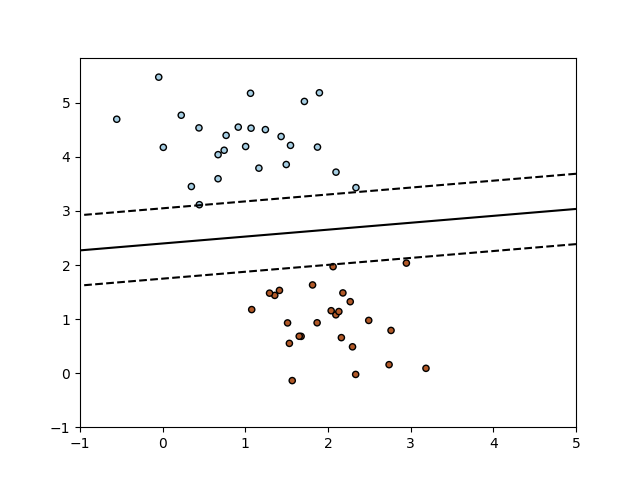>>> from sklearn.linear_model import SGDClassifier
>>> X = [[0., 0.], [1., 1.]]
>>> y = [0, 1]
>>> clf = SGDClassifier(loss="hinge", penalty="l2")
>>> clf.fit(X, y)
SGDClassifier(alpha=0.0001, average=False, class_weight=None,
early_stopping=False, epsilon=0.1, eta0=0.0, fit_intercept=True,
l1_ratio=0.15, learning_rate='optimal', loss='hinge',
max_iter=1000, n_iter_no_change=5, n_jobs=None, penalty='l2',
power_t=0.5, random_state=None, shuffle=True, tol=0.001,
validation_fraction=0.1, verbose=0, warm_start=False)


>>> clf.predict([[2., 2.]])
array()


SGD 通过训练数据来拟合一个线性模型。成员 coef_ 保存模型参数:

>>> clf.coef_
array([[ 9.9...,  9.9...]])


>>> clf.intercept_
array([-9.9...])


>>> clf.decision_function([[2., 2.]])
array([ 29.6...])


• loss="hinge": (soft-margin) linear Support Vector Machine （（软-间隔）线性支持向量机），
• loss="modified_huber": smoothed hinge loss （平滑的 hinge 损失），
• loss="log": logistic regression （logistic 回归），
• and all regression losses below（以及所有的回归损失）。

>>> clf = SGDClassifier(loss="log").fit(X, y)
>>> clf.predict_proba([[1., 1.]])
array([[ 0.00...,  0.99...]])


• penalty="l2": L2 norm penalty on coef_.
• penalty="l1": L1 norm penalty on coef_.
• penalty="elasticnet": Convex combination of L2 and L1（L2 型和 L1 型的凸组合）; (1 - l1_ratio) * L2 + l1_ratio * L1.

SGDClassifier 通过利用 “one versus all” （OVA）方法来组合多个二分类器，从而实现多分类。对于每一个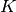类, 可以训练一个二分类器来区分自身和其他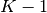个类。在测试阶段，我们计算每个分类器的 confidence score（置信度分数）（也就是与超平面的距离），并选择置信度最高的分类。下图阐释了基于 iris（鸢尾花）数据集上的 OVA 方法。虚线表示三个 OVA 分类器; 不同背景色代表由三个分类器产生的决策面。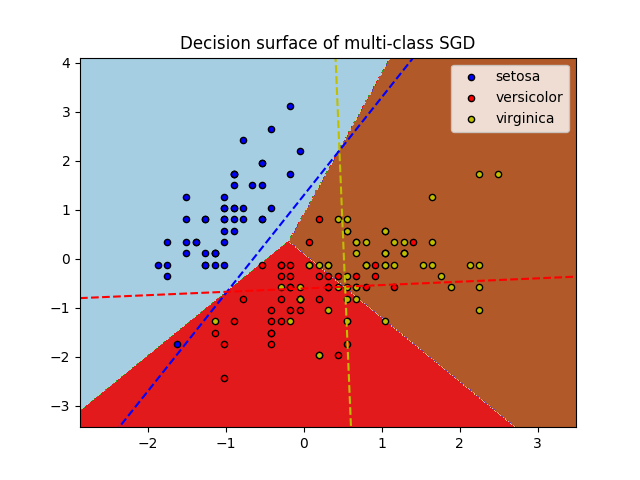SGDClassifier 通过拟合参数 class_weightsample_weight 来支持 weighted classes （加权类）和 weighted instances（加权实例）。更多信息请参照下面的示例和 SGDClassifier.fit 的文档。

SGDClassifier 支持 averaged SGD (ASGD)。Averaging（均值化）可以通过设置 average=True 来启用。AGSD 工作原理是在普通 SGD 的基础上，对每个样本的每次迭代后的系数取均值。当使用 ASGD 时，学习速率可以更大甚至是恒定，在一些数据集上能够加速训练过程。

## 1.5.2. 回归

SGDRegressor 类实现了一个简单的随机梯度下降学习例程，它支持用不同的损失函数和惩罚来拟合线性回归模型。 SGDRegressor 非常适用于有大量训练样本（>10.000)的回归问题，对于其他问题，我们推荐使用 RidgeLasso ，或 ElasticNet

• loss="squared_loss": Ordinary least squares（普通最小二乘法）,
• loss="huber": Huber loss for robust regression（Huber回归）,
• loss="epsilon_insensitive": linear Support Vector Regression（线性支持向量回归）.

Huber 和 epsilon-insensitive 损失函数可用于 robust regression（鲁棒回归）。不敏感区域的宽度必须通过参数 epsilon 来设定。这个参数取决于目标变量的规模。

SGDRegressor 支持 ASGD（平均随机梯度下降） 作为 SGDClassifier。 均值化可以通过设置 average=True 来启用。

## 1.5.3. 稀疏数据的随机梯度下降

• 由于在截距部分收敛学习速率的差异，稀疏实现与密集实现相比产生的结果略有不同。

scipy.sparse 支持的格式中，任意矩阵都有对稀疏数据的内置支持方法。但是，为了获得最高的效率，请使用 scipy.sparse.csr_matrix 中定义的 CSR 矩阵格式.

## 1.5.4. 复杂度

SGD 主要的优势在于它的高效性，对于不同规模的训练样本，处理复杂度基本上是线性的。假如 X 是 size 为 (n, p) 的矩阵，训练成本为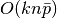，其中 k 是迭代次数，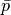是每个样本非零特征的平均数。

## 1.5.5. 停止判据

SGDClassifierSGDRegressor 类提供了两个判据，当达到给定的收敛水平时停止算法:

• early_stopping = True时,输入数据分为训练集和验证集。该模型在训练集拟合,停止判据是基于验证集上的预测分数。改变参数validation_fraction可以调整验证集的大小。

• early_stop = False时，模型对整个输入数据进行拟合，停止判据基于整个输入数据上的目标函数来计算。

## 1.5.6. 实用小贴士

• 随机梯度下降法对 feature scaling （特征缩放）很敏感，因此强烈建议您缩放您的数据。例如，将输入向量 X 上的每个特征缩放到 [0,1] 或 [- 1，+1]， 或将其标准化，使其均值为 0，方差为 1。请注意，必须将 相同 的缩放应用于对应的测试向量中，以获得有意义的结果。使用 StandardScaler能很容易做到这一点：
from sklearn.preprocessing import StandardScaler
scaler = StandardScaler()
scaler.fit(X_train) # Don’t cheat - fit only on training data
X_train = scaler.transform(X_train)
X_test = scaler.transform(X_test) # apply same transformation to test data


## 1.5.7. 数学描述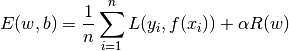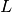的不同选择产生不同的分类器，例如

• Hinge: (软-间隔) 支持向量机。
• Log: Logistic 回归。
• Least-Squares: 岭回归。
• Epsilon-Insensitive: (软-间隔) 支持向量回归。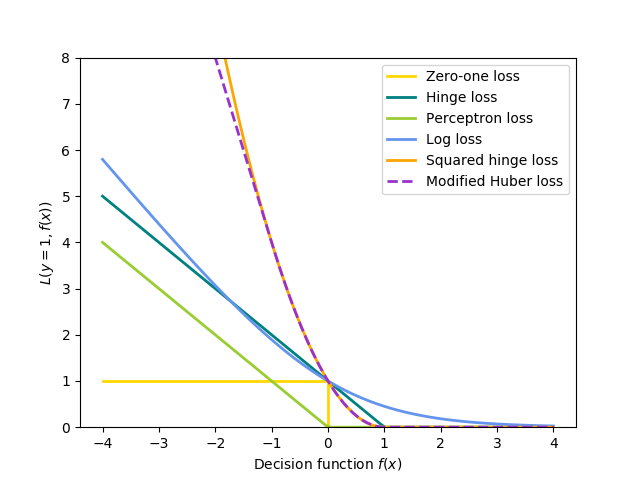• L2 norm: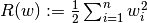,
• L1 norm: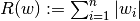, 这导致了稀疏解。
• Elastic Net: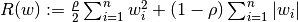, l2和l1的凸组合, 其中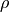1 - l1_ratio给出.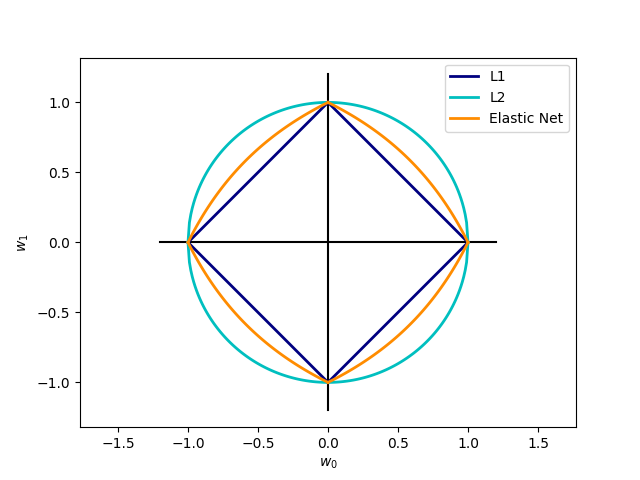### 1.5.7.1. SGD

SGDClassifier 类实现了一个 first-order SGD learning routine （一阶 SGD 学习程序）。 算法在训练样本上遍历，并且对每个样本根据由以下式子给出的更新规则来更新模型参数。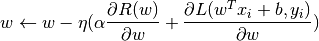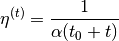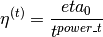• 成员 coef_ holds the weights（控制权重）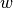• 成员 intercept_ holds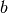## 1.5.8. 实现细节

SGD 的实现受到了 Léon Bottou Stochastic Gradient SVM 的影响。类似于 SvmSGD，权重向量表达为一个标量和一个向量的内积，这样保证在使用L2正则项时可以高效更新权重。 在 sparse feature vectors （稀疏特征向量）的情况下， intercept （截距）是以更小的学习率（乘以 0.01）更新的，这导致了它的更新更加频繁。训练样本按顺序选取并且每处理一个样本就要降低学习速率。我们采用了 Shalev-Shwartz 等人2007年提出的的学习速率设定方案。 对于多类分类，我们使用了 “one versus all” 方法。 我们在 L1 正则化（和 Elastic Net ）中使用 Tsuruoka 等人2009年提出的 truncated gradient algorithm （截断梯度算法）。代码是用 Cython 编写的。